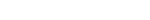%

%

What is percentage of increase or decrease

%

The percentage calculator is an extremely easy to use tool which can perform both simple and complex calculations with a click of a button. The tool is very user-friendly and can calculate percentage based on the criteria specified by the user. You only need to enter values in the above given first two input fields and press calculate button, the answer will be shown in the last input field.

As you can see, there are 3 percentage calculators given above so pick the one which fits your need.

# What is percentage?

The percentage is an easiest way with which a number, ratio, or fraction can be expressed. It is one of the most commonly used method to express probabilities of profits or loss in any kind of exchange or investment where the numbers are involved. The sign used to express percentage is %

# How to calculate value from percentage?

In order to calculate the value from the percentage, we generally use the formula V = P% * X

For instance, to calculate 30% of 100, we can use the above formula. Lets have a look over the steps involved in the calculation process.

1. We have P as 30% and X in this case, is 100.
2. Using those values in the formula, we have V = 30% * 100
3. After converting 30% into decimal form, we have 0.30
4. Now, the value V = 0.30 * 100 =30
5. V = 30
6. Hence, 30% of 100 is 30.

# Using First Percentage Calculator

We need to input '30' in the first field and '100' in the second field. After clicking calculate button we will get 30 in the third field, which is the same value we got from above calculation.

# How to convert value to percentage?

The simplest formula of calculating the percentage of a number is given below. P stands for percentage. It can be read as X1 is P percentage of X2.

For instance, if you want to find out 50 is what percentage of 300, we use the following given steps.

1. Using this formula, , we have as 50 and as 300.
2. After substituting the values in the formula, we have
3. 50 divided by 300 is equal to 0.1667
4. The output 0.1667 is in decimal form. We need to multiply it by 100 to convert it to percentage.
5. Therefore,
6. Hence, 50 is 16.67% of 300.

# Using Second Percentage Calculator

We need to input '50' in the first field and '300' in the second field. After clicking calculate button we will get 16.67% in the third field, which is the same value we got from above calculation.

# How to calculate increase/decrease in percentage?

It’s very common to calculate profit or loss in in terms of percentage. The easiest way to calculate percentage increase or decrease is by computing the difference between the two values and displaying it relative to the initial value. The inputs are taken absolute values and returned percentage can have negative or positive sign before it.

# Using Third Percentage Calculator

To calculate the Percentage Decrease, we need to input 100 (assume it as 100\$) in the first field and 50 (assume it as 50\$) in the second field. The calculator will give us an output of -50%, which means that there has been 50% decrease in the price from 100\$.

To calculate the Percentage increase, we need to input 200 (assume it as 200\$) in the first field and 300 (assume it as 300\$) in the second field. The calculator will give us an output of 50%, which means that there has been 50% increase in the price from 200\$.

# Usage of Third Percentage Calculator

Such percentage increase or decrease calculations are very import if you are in finance sector or person who invests regularly and wants to calculate profit or loss after investment.

• If you are a regular shopper and interested in discounts shown in banners in various shops, then you can easily calculate how much money you are saving after those discounts.
• If you are an accountant then it can help you in performing daily calculations of such as tax, interest, inflation, etc.

Disclaimer:We make a reasonable effort in making sure that calculation results are as accurate as possible, but we cannot guarantee that. Before using any details provided here, you must validate its correctness from other reliable sources on internet.